# Flowers 2

Cha cruz has a garden. The ratio roses to tulips is 2 : 5, the ratio of roses to orchids is 7 : 6. Cha cruz wonders what the ratio of tulips to orchids is. If Cha cruz has 183 plants, how many of each kind are there?

r =  42
t =  105
o =  36
p =  35:12

### Step-by-step explanation:

$t=105=105$
$o=36=36$
$p=\frac{t}{o}=\frac{105}{36}=\frac{35}{12}=2\frac{11}{12}\approx 2.9167=35:12$Did you find an error or inaccuracy? Feel free to write us. Thank you!Tips to related online calculators
Check out our ratio calculator.
Do you have a linear equation or system of equations and looking for its solution? Or do you have a quadratic equation?

## Related math problems and questions:

• Garden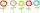The garden grows 312 roses and 389 tulips. Which flowers are more? How many?
• Crocuses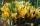The garden grows daffodils, crocuses, and roses. 1400 daffodils, crocuses are 462 more, and roses are 156 more than crocuses. How many are all the flowers in the garden?
• Gear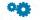Two gears, fit into each other, has transfer 2:3. Centres of gears are spaced 82 cm. What are the radii of the gears?
• Tulips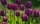Of the 120 tulips in the garden, 45 are red, 66 are yellow, the rest are brindle. What percentage of tulips in the garden are brindle?
• Ratio to total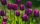7:12 is the ratio of orchids to tulips. What is the ratio of the number of orchids to the total number of flowers in the garden?
• Sweets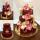3 chocolate and 7 cakes cost 85, - CZK. 2 chocolates and 6 cakes cost 86, - CZK. How much is 5 chocolates and 9 cakes? I wonder how to get the result, but only by logic without the use of a system of equations
• Ratio6 numbers are in the ratio 1:5:1:5:5:5. Their sum is 242. What are the numbers?
• Jane plantsJane plants flowers in the garden. If she planted 12 every hour instead of 9 flowers, she would finish with the job an hour earlier. How many flowers does she plant?
• Roses and tulipsAt the florist are 50 tulips and 5 times fewer roses. How many flowers are in the flower shop?
• Numbers at ratio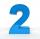The two numbers are in a ratio 3:2. If we each increase by 5 would be at a ratio of 4:3. What is the sum of original numbers?
• Neighborhood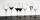I have 7 cups: 1 2 3 4 5 6 7. How many opportunities of standings cups are there if 1 and 2 are always neighborhood?
• Tulips and daffodils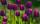Two hundred twenty tulips and daffodils are planted in the flowerbed. One-third of all tulips and one-sixth of all daffodils equals the number of all tulips. How many tulips and how many daffodils?
• The ratio 3The ratio of male pupils to female pupils in Gymnasium is 7:9. If there are 512 pupils, how many pupils are male?
• School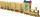School attend 792 children, boys and girls ratio is 4:5. How many more girls go to school (than boys)?
• Columns of two and threeWhen students in one class stand in columns of two, there is none left. When he stands in columns of three, there is one student left. There are 5 more double columns than three columns. How many students are in the class?
• Division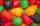Three siblings Helena, Oliver and George split the bag with candies on merit in the ratio 6:1:4. How many candies should each get if in bag were 88?
• 1200 screws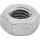1200 screws are to be divided into 3 groups so that in the 1st group there are 300 screws more than in the 2nd group and in the 2nd group 150 screws less than in the 3rd group. How many screws will be in each group?